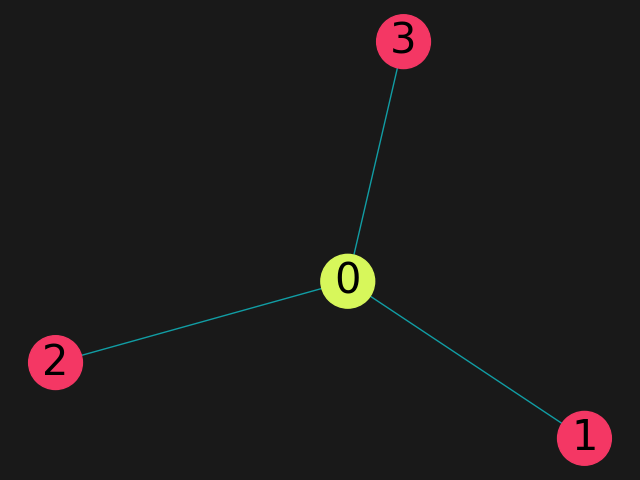Applications

# Quantum Computing Applications - Optimization

22
March
,
2022

Optimization problems are found in numerous industries. Here are just a few examples:

• Portfolio optimization. A money manager has a certain amount of money to invest. What is the optimal allocation of these funds?
• Route optimization. A FedEx truck needs to deliver 50 packages. What is the optimal route to deliver the packages?
• Airport security. An airport wishes to install security cameras to watch over all corners in the airport. What is the optimal arrangement of cameras that achieves this?

Each optimization has some definition of what constitutes an optimal solution, and a set of constraints. Let’s explore the optimal solution and some possible constraints for each example:

Portfolio optimization. The money manager might seek the highest return with the lowest risk. The constraint might  be, for instance, not investing more than 5% of the portfolio in any single asset.

Route optimization.The FedEx truck might want to complete the deliveries in the minimum amount of time. Alternative definitions of the optimal solution, instead of the shortest delivery time, might be the lowest-cost route, considering fuel and toll charges, or the route that generates the lowest carbon footprint. The constraint might be not to travel on certain residential roads before 8 AM.

Airport security. The airport might wish to cover all corridors with the smallest number of cameras. A constraint might be that there must be at least one camera in each concourse.

It’s easy to see how optimization problems can become exceptionally complex. The FedEx truck with 50 stops has about 30 vigintillion
(that’s 3 x 10^64) possible options. Even if the optimization can be completed in a reasonable amount of time, it might need to be redone at a moment’s notice: There is a major traffic jam on the route, the price of an asset has dropped making it more attractive for purchase, etc.

But when problems are hard, solving them could provide a big reward. A logistics company that saves 15% on fuel costs because of optimized routing can increase its profits or grow its market share. An optimal portfolio is good for the customer, the portfolio manager, and her employer.

## Here's how it looks with Classiq:

Take the airport security problem. This type of problem is known as a “max vertex cover” problem.

The user specifies the configuration or floor plan of the airport and the number of cameras they want to use, and Classiq’s synthesis engine sets up the mathematics of the problem.

In technical terms, the user provides a weighted edge graph and size of the vertex cover. Classiq provides a function that defines the problem, constraints, and solution. The problem is then solved using QAOA (Quantum Approximate Optimization Algorithm). This is a hybrid algorithm - a quantum portion (quantum cost function encoding the solution or the expectation value we want to optimize, a quantum mixer function to explore each possible solution, a quantum ansatz or parametrized initial state), and a classical optimization portion (in our case, we use the Pyomo package).

Here we specify a star graph with three outer nodes like pictured below. For convenience, we marked each node with a number. If we only have one camera, what position would cover all areas of the airport?


import networkx as nx
import pyomo.core as pyo
from classiq import combinatorial_optimization
from classiq_interface.combinatorial_optimization.preferences import QAOAPreferences
from classiq_interface.combinatorial_optimization.solver_types import QSolver

def mvc(graph: nx.Graph, k: int) -> pyo.ConcreteModel:
model = pyo.ConcreteModel()
model.x = pyo.Var(graph.nodes, domain=pyo.Binary)
model.amount_constraint = pyo.Constraint(expr=sum(model.x.values()) == k)

def obj_expression(model):
return sum((1 - model.x[i]) * (1 - model.x[j]) for i, j in graph.edges)

model.cost = pyo.Objective(rule=obj_expression, sense=pyo.minimize)

return model

G = nx.star_graph(3)
mvc_model = mvc(G, 1)

qaoaP = QAOAPreferences(qsolver=QSolver.QAOAMixer)
mvc_problem = combinatorial_optimization.CombinatorialOptimization(model=mvc_model,
qaoa_preferences=qaoaP)
qc = mvc_problem.generate()
qc.show_interactive()

open("MVC_interactive_circuit.html", "w").write(qc.interactive_html)

result = mvc_problem.solve()
print(result)


Once we generate the circuit, an interactive circuit pops up in a new window. The circuit’s structure is clearly seen, and we can dive deeper into any part of the circuit by clicking the plus icon in the top left corner.

interactive visualizationThere was some error with the script

When we execute this circuit with the QAOA and print the results, we get this solution (chosen because the algorithm works to minimize the ‘cost’ function).

The list corresponds to the potential camera locations, so position 0, the center node, should have a camera.Here’s what the solution looks like with another graph. Can you confirm there are no corners left unseen?